Question

Question 3
<
B0/2 pts
A friend has a 81% average before the final exam for a course. That score includes everything but
the final, which counts for 20% of the course grade.
What is the minimum score would your friend would need on the final to earn a 75% for the course?
Give answers accurate to at least one decimal place.
Question Help: Video Message instructor
Submit Question
o
earch

1.The best course grade your friend can earn is 0.848 = 84.8%.

The minimum score would your friend would need on the final to earn a 75% for the course is of 0.51 = 51%.

Step-by-step explanation:

This is a weighed average problem, in which we multiply each grade by its weight.

We have that:

In 80% of the course, the friend has a grade of 81%.

In the other 20%, he will have x.

This will happen if he earns 100% = 1 on the final test. So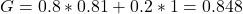The best course grade your friend can earn is 0.848 = 84.8%.

What is the minimum score would your friend would need on the final to earn a 75% for the course?

This is x, when the grade is 0.75. So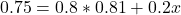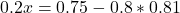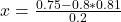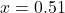The minimum score would your friend would need on the final to earn a 75% for the course is of 0.51 = 51%.